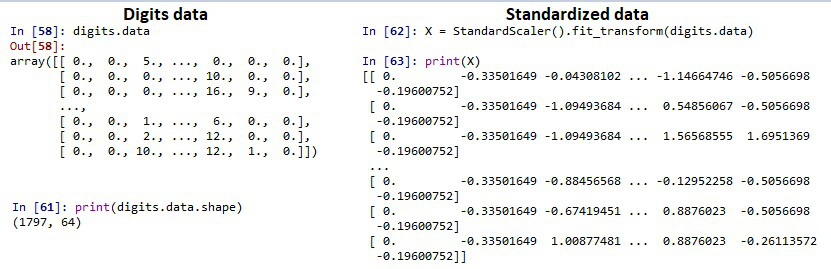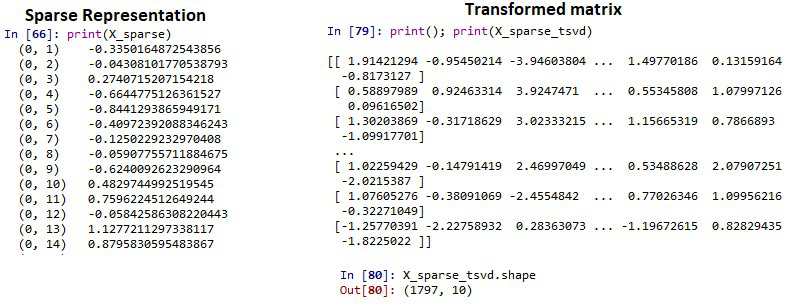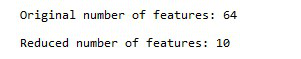Open in App
Not now

# How to reduce dimensionality on Sparse Matrix in Python?

• Last Updated : 19 Sep, 2022

A matrix usually consists of a combination of zeros and non-zeros. When a matrix is comprised mostly of zeros, then such a matrix is called a sparse matrix. A matrix that consists of maximum non-zero numbers, such a matrix is called a dense matrix. Sparse matrix finds its application in high dimensional Machine learning and deep learning problems. In other words, when a matrix has many of its coefficients as zero, such a matrix is said to be sparse.

The common area where we come across such sparse dimensionality problems is

• Natural Language Processing – It is obvious that most of the vector elements of the document will be 0s in language models
• Computer Vision – Sometimes an image can be occupied by similar color (eg, white which can be a background) that doesn’t give us any useful information.

In such cases, we cannot afford to have a matrix of the large dimensional matrix, as it can increase the time and space complexity of the problem, so it is recommended to reduce the dimensionality of the sparse matrix. In this article let us discuss the implementation of how to reduce the dimensionality of the sparse matrix in python

The dimensionality of the sparse matrix can be reduced by first representing the dense matrix as a Compressed sparse row representation in which the sparse matrix is represented using three one-dimensional arrays for the non-zero values, the extents of the rows, and the column indexes. Then, by using scikit-learn’s TruncatedSVD, it is possible to reduce the dimensionality of the sparse matrix.

Example:

First load the inbuilt digits dataset from the scikit-learn package, Standardize each data point using standardscaler. Represent the Standardized matrix in its sparse form using csr_matrix as shown. Now import the TruncatedSVD from sklearn and specify the no. of dimensions required in the final output Finally check for the shape of the reduced matrix

## Python3

 `from` `sklearn.preprocessing ``import` `StandardScaler` `from` `sklearn.decomposition ``import` `TruncatedSVD` `from` `scipy.sparse ``import` `csr_matrix` `from` `sklearn ``import` `datasets` `from` `numpy ``import` `count_nonzero`   `# load the inbuild digits dataset` `digits ``=` `datasets.load_digits()`   `print``(digits.data)`   `# shape of the dense matrix` `print``(digits.data.shape)`   `# standardizing the data points` `X ``=` `StandardScaler().fit_transform(digits.data)` `print``(X)`   `# representing in CSR form` `X_sparse ``=` `csr_matrix(X)` `print``(X_sparse)`   `# specify the no of output features` `tsvd ``=` `TruncatedSVD(n_components``=``10``)`   `# apply the truncatedSVD function` `X_sparse_tsvd ``=` `tsvd.fit(X_sparse).transform(X_sparse)` `print``(X_sparse_tsvd)`   `# shape of the reduced matrix` `print``(X_sparse_tsvd.shape)`

Output:Code:

Let us cross verify the original dimension and transformed dimension

## Python3

 `print``(``"Original number of features:"``, X.shape[``1``])` `print``(``"Reduced number of features:"``, X_sparse_tsvd.shape[``1``])`

Output:My Personal Notes arrow_drop_up
Related Articles